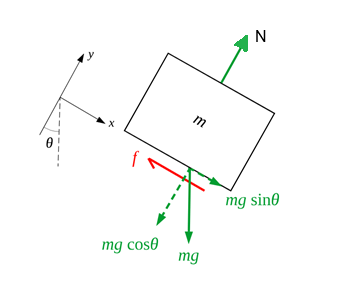# Problem: To determine the coefficients of friction between rubber and various surfaces, a student uses a rubber eraser and an incline. In one experiment, the eraser begins to slip down the incline when the angle of inclination is 36.0° and then moves down the incline with constant speed when the angle is reduced to 30.0°. From these data, determine the coefficients of (a) static friction, and (b) kinetic frictionfor this experiment.

###### FREE Expert Solution

This problem requires us to determine the static and kinetic coefficients of friction.

Whenever there’s an inclined plane problem where something “starts to slip” or “begins sliding”, this involves the Critical Angle, which we have an easy equation for. Let’s check it out….

1. Draw a free-body diagram of the eraser.
2. Resolve the weight component and write the ΣF=ma equations.
3. Solve for the target variables.

Step 1: We need to represent the forces acting on the eraser in a free-body diagram as shown below.From the free-body diagram, the x component of the gravitational force, mg sinθ, acts on the eraser downslope while friction force, f, acts in the opposite direction.

is the normal force between the eraser and the incline.

The friction force is equal to the product of the normal force and the coefficient of friction, $\overline{){\mathbit{f}}{\mathbf{=}}{\mathbit{\mu }}{\mathbit{N}}}$

For a stationary body, we use the static coefficient, µs, of friction. Static friction, fs, is equal to the maximum force applied on an object before it starts moving.

For an object in motion, we use the kinetic coefficient, µk, of friction. Kinetic friction, fk, is equal to the force required to keep an object moving with constant speed.

We are going to break the problem into two parts: static coefficient of friction and kinetic coefficient of friction.

Step 2: Write the ΣF=ma equations

(a) Determining the static coefficient of friction

To find the coefficient of friction, we determine the magnitude of forces acting on the eraser. The x component of gravitational force mg sinθ,  acts on the eraser down the incline while the frictional force, ${\mathbit{f}}{\mathbf{=}}{\mathbit{\mu }}{\mathbit{N}}$, acts on the eraser up the slope.

80% (249 ratings)###### Problem Details

To determine the coefficients of friction between rubber and various surfaces, a student uses a rubber eraser and an incline. In one experiment, the eraser begins to slip down the incline when the angle of inclination is 36.0° and then moves down the incline with constant speed when the angle is reduced to 30.0°. From these data, determine the coefficients of

(a) static friction, and

(b) kinetic friction

for this experiment.